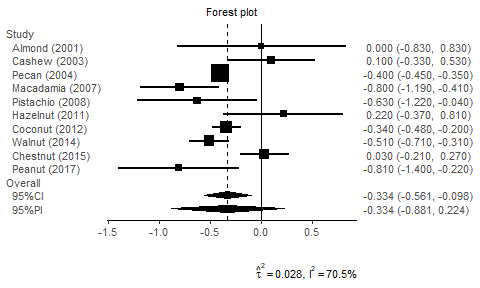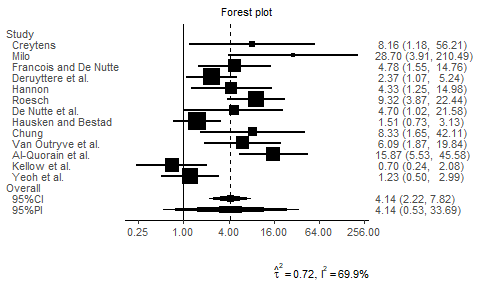# Introduction to the pimeta package

#### 2019-09-17

The pimeta package is easy. Load your data and then pass it the pima function!

library("pimeta")
library("ggplot2")
## Warning: package 'ggplot2' was built under R version 3.6.1
data(sbp, package = "pimeta")

# a parametric bootstrap prediction interval
piboot <- pima(
y        = sbp$y, # effect size estimates se = sbp$sigmak, # within studies standard errors
B        = 25000,      # number of bootstrap samples
seed     = 14142135,   # random number seed
parallel = 4           # multi-threading
)
piboot
##
## Prediction & Confidence Intervals for Random-Effects Meta-Analysis
##
## A parametric bootstrap prediction and confidence intervals
##  Heterogeneity variance: DerSimonian-Laird
##  Variance for average treatment effect: Hartung (Hartung-Knapp)
##
## No. of studies: 10
##
## Average treatment effect [95% prediction interval]:
##  -0.3341 [-0.8807, 0.2240]
##  d.f.: 9
##
## Average treatment effect [95% confidence interval]:
##  -0.3341 [-0.5613, -0.0985]
##  d.f.: 9
##
## Heterogeneity measure
##  tau-squared: 0.0282
##  I-squared:  70.5%
plot(piboot, base_size = 10, studylabel = sbp$label)Several type of methods ("HTS", "HK", "SJ", …) are supported. # Higgins-Thompson-Spiegelhalter prediction interval pima(sbp$y, sbp$sigmak, method = "HTS") ## ## Prediction & Confidence Intervals for Random-Effects Meta-Analysis ## ## Higgins-Thompson-Spiegelhalter prediction and confidence intervals ## Heterogeneity variance: DerSimonian-Laird ## Variance for average treatment effect: approximate ## ## No. of studies: 10 ## ## Average treatment effect [95% prediction interval]: ## -0.3341 [-0.7598, 0.0917] ## d.f.: 8 ## ## Average treatment effect [95% confidence interval]: ## -0.3341 [-0.5068, -0.1613] ## d.f.: 9 ## ## Heterogeneity measure ## tau-squared: 0.0282 ## I-squared: 70.5% The convert_bin() function converts binary outcome data to effect size estimates and within studies standard errors vectors. A data set of 13 placebo-controlled trials with cisapride that was reported by Hartung and Knapp (Stat Med., 2001, doi:10.1002/sim.1009) was analyzed below. Estimated values on the logarithmic scale can be back-transformed to the original scale with the trans option (in print and plot). m1 <- c(15,12,29,42,14,44,14,29,10,17,38,19,21) n1 <- c(16,16,34,56,22,54,17,58,14,26,44,29,38) m2 <- c( 9, 1,18,31, 6,17, 7,23, 3, 6,12,22,19) n2 <- c(16,16,34,56,22,55,15,58,15,27,45,30,38) dat <- convert_bin(m1, n1, m2, n2, type = "logOR") head(dat, n = 3) ## y se ## 1 2.098986 0.9847737 ## 2 3.357026 1.0165653 ## 3 1.565232 0.5747840 pibin <- pima(dat$y, dat$se, seed = 2236067, parallel = 4) print(pibin, digits = 3, trans = "exp") ## ## Prediction & Confidence Intervals for Random-Effects Meta-Analysis ## ## A parametric bootstrap prediction and confidence intervals ## Heterogeneity variance: DerSimonian-Laird ## Variance for average treatment effect: Hartung (Hartung-Knapp) ## ## No. of studies: 13 ## ## Average treatment effect [95% prediction interval]: ## 4.141 [0.533, 33.692] ## d.f.: 12 ## Scale: exponential transformed ## ## Average treatment effect [95% confidence interval]: ## 4.141 [2.224, 7.820] ## d.f.: 12 ## Scale: exponential transformed ## ## Heterogeneity measure ## tau-squared: 0.718 ## I-squared: 69.9% binlabel <- c( "Creytens", "Milo", "Francois and De Nutte", "Deruyttere et al.", "Hannon", "Roesch", "De Nutte et al.", "Hausken and Bestad", "Chung", "Van Outryve et al.", "Al-Quorain et al.", "Kellow et al.", "Yeoh et al.") plot(pibin, digits = 2, base_size = 10, studylabel = binlabel, trans = "exp")Save a plot to PNG: png("forestplot.png", width = 500, height = 300, family = "Arial") plot(piboot, digits = 2, base_size = 18, studylabel = sbp$label)
dev.off()

or

p <- plot(piboot, digits = 2, base_size = 10, studylabel = sbp\$label)
ggsave("forestplot.png", p, width = 5, height = 3, dpi = 150)